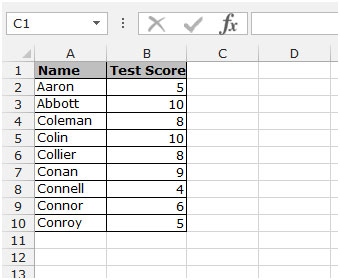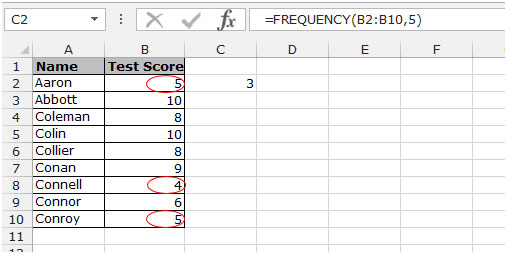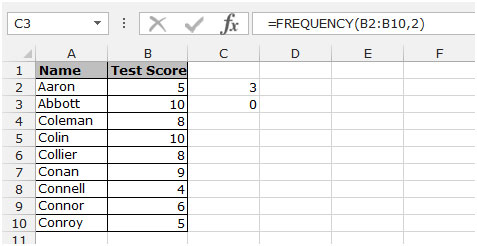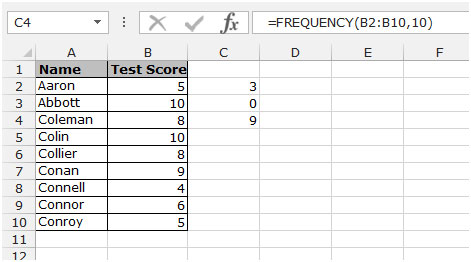# "FREQUENCY" Function in Microsoft Excel 2010

In this article we will learn how and where we can use the Frequency function in Microsoft Excel 2010 and 2013.

FREQUENCY: This function calculates how often values occur within a range of values then returns a vertical array of numbers having one more element than Bins array.

The syntax of FREQUENCY function:-

=FREQUENCY(data_array,bins_array)

Let’s take an example and understand how and where we can use Frequency function in Microsoft Excel 2010 and 2013.

To create the Frequency formula and array enter it into Excel

Example 1: We have data in the range A1:B10. In which column A contains name and column B contains test score.Let’s say in the data we want to check the frequency of number 5, to check the frequency follow the below given steps:-

• Select the cell C2 and write the formula.
• =FREQUENCY(B2:B10,5)press enter.
• The function will return 3, it means 3 numbers are there till the 5 number.Let’s say in the data, to check the frequency of number 2 follow the below given steps:-

• Select the cell C3 and write the formula.
• =FREQUENCY(B2:B10,2) press enter.
• The function will return 0, it means 0 numbers are there till the 2 number.Let’s say in the data to check the frequency of number 10,Select the cell C4 and write the formula.

• =FREQUENCY(B2:B10,10) press enter.
• The function will return 9, it means 9 numbers are there till the 10 number.This is the way to check frequency in data by using the Frequency function in Microsoft Excel 2010 and 2013.

## Users are saying about us...

1.Amazing

Terms and Conditions of use

The applications/code on this site are distributed as is and without warranties or liability. In no event shall the owner of the copyrights, or the authors of the applications/code be liable for any loss of profit, any problems or any damage resulting from the use or evaluation of the applications/code.